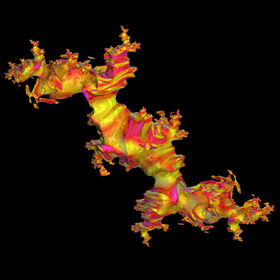## Archive for the 'Mandelbrot' Category

18
Aug
09

### 3D Mandelbrot Set Pickover StalksPickover Stalks are cross-shaped orbit traps invented by Clifford Pickover. See also my 2D Pickover stalks fractal.

Advertisements
18
Aug
09

### 3D Mandelbrot Set Orbit TrapOrbit trapping is a popular technique for mapping arbitrary shapes to a fractal. This 3D orbit trap is shaped like a sphere. See also my 2D orbit trap fractals.

30
Jul
09

### 4D “Mandy Cousin” Julia and Mandelbrot Sets

{x,y,z,w}2 = {x2-y2-z2+w2, 2(xy+zw), 2(xz+yw), 2(xw+yz)}
David Makin suggested this formula to me for squaring a 4D hypercomplex number.

30
Jul
09

### 4D Julia and Mandelbrot Sets VariationsJulia : c={-0.2,0.8,0,0}, imax=12 {x,y,z,w}2 = {x2-y2-z2-w2, 2(xy+zw), 2(xz-yw), 2(xw+yz)} Here is another formula for squaring a 4D hypercomplex number that David Makin suggested to me.
30
Jul
09

### 4D Bicomplex Mandelbrot Set

{x,y,z,w}2 = {x2-y2-z2+w2, 2(xy-zw), 2(xz-yw), 2(xw+yz)}
This “Tetrabrot” reminds me of a Bismuth crystal.

Here is some Mathematica code for the minibrot:
```(* runtime: 2.6 minutes, increase n for higher resolution *) n = 100; Norm[x_] := x.x; Square[{x_, y_, z_, w_}] := {x^2 - y^2 - z^2 + w^2, 2 (x y - z w), 2 (x z - y w), 2 (x w + y z)}; Mandelbrot4D[qc_] := Module[{q = {0, 0, 0, 0}, i = 0}, While[i < 20 && Norm[q] < 4, q = Square[q] + qc; i++]; i]; image = Table[z = 0.06; While[z >= 0 && Mandelbrot4D[{x, y, z, 0}] < 20, z -=0.12/n]; If[z < 0, -0.06, z], {y, -0.06, 0.06, 0.12/n}, {x, -1.82, -1.7, 0.12/n}]; ListDensityPlot[image, Mesh -> False, Frame -> False, PlotRange -> {-0.02, 0.06}]```

24
Jul
09

### 4D “Roundy” Mandelbrot Set

{x,y,z,w}2 = {x2-y2-z2-w2, 2(xy+zw), 2(xz+yw), 2(xw+yz)}
This formula for squaring a 4D hypercomplex number is probably the most popular method for creating 3D Mandelbrot sets. Here is some Mathematica code:
```(* runtime: 1.2 minutes, increase n for higher resolution *) n = 100; Norm[x_] := x.x; Square[{x_, y_, z_, w_}] := {x^2 - y^2 - z^2 - w^2, 2 (x y + z w), 2 (x z + y w), 2 (x w + y z)}; Mandelbrot4D[qc_] := Module[{q = {0, 0, 0, 0}, i = 0}, While[i < 24 && Norm[q] < 4, q = Square[q] + qc; i++]; i]; image = Table[z = 1.5; While[z >= 0 && Mandelbrot4D[{x, y, z, 0}] < 24, z -= 3/n]; If[z < 0, -1.5, z], {y, -1.5, 1.5, 3/n}, {x, -2.0, 1.0, 3/n}]; ListDensityPlot[image, Mesh -> False, Frame -> False, PlotRange -> {-0.1,1.5}]```

07
Jul
09

### 4D Quaternion Mandelbrot Set

{x,y,z,w}2 = {x2-y2-z2-w2, 2xy, 2xz, 2xw}
Quaternions are 4D hypercomplex numbers, discovered in 1843 by Sir William Rowan Hamilton. They are mathematically elegant, but unfortunately, they produce axisymmetric results when used to calculate the 3D Mandelbrot set.

Link: Quat Minibrot – by David Makin

## Welcome !

You will find here some of my favorite hobbies and interests, especially science and art.

I hope you enjoy it!

Subscribe to theRSS feed to stay informed when I publish something new here.

I would love to hear from you! Please feel free to send me an email : bugman123-at-gmail-dot-com

## Recent CommentsBerna Blalack on Magnetic Pendulum Strange…Daan on Magnetic Pendulum Strange…Sebastian Schepis on Diffusion Limited Aggregation…mohammad_andito on CFM56-5 Turbofan Jet Engi…SasQ on Magnetic Field of a Solen… OUPblog » Blog… on Diamond Light Dispersion Complex Roots on Polynomial Roots Joukowski airfoils |… on Joukowski AirfoilKarim Alame on Flapping WingREJISH J on Joukowski Airfoil SOLINOID | Materials… on Magnetic Field of a Solen…Emanuele on 4D “Squarry” Julia…Emanuele on Hydrogen Electron Orbital Prob…Tim on Mandelbrot Set Pickover S…khankasi1 on 4D Quaternion Mandelbrot …

• 561,072 hits
Advertisements